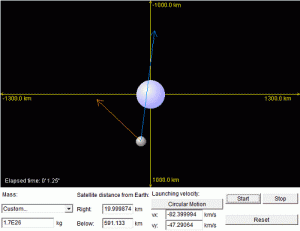# Planet Orbit SimulationThis Java applet simulates the motion of a satellite, orbiting around another planet. You can interactively change the launch velocity of the satellite to explore different orbital path that it will produce. The motion of the satellite is calculated as accurate as possible using Newton’s Law of Motion and Newton’s Law of Gravitation. The applet can even automatically calculates the launch velocity of the satellite that will produce a circular orbit based on its current position. The calculation is done based on Kepler’s Law. The small motion of the central planet is neglected in this applet. I developed this Javaapplet mainly to learn and explore the pattern of an orbiting mass around a central body in a more interesting and interactive manner.